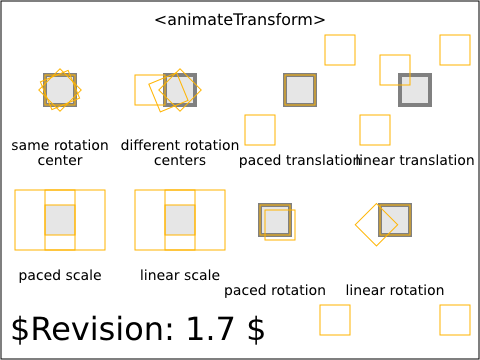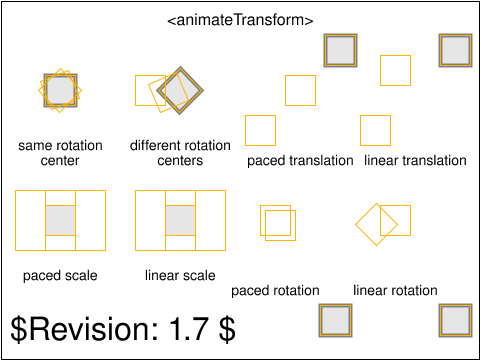Tests that contain the draft-watermark are under development and may be incorrectly testing a feature.

# animate-elem-82-t.svg## Pass Criteria

The test is passed if the lightgray rectangles are exactly in the positions indicated by each of the orange rectangles when they are shown with a thick stroke. If any part of the lightgray rectangles are outside the thick stroked orange rectangles then the test has failed.

## Test Description

This test demonstrates validates the operation of animateTransform with regards to the rotation center and with regards to paced animation.

The following descriptions describe the various animations, going top bottom, left to right. For each animation, orange rectangle markers show the expected position for the animated rectangle halfway through the animation. The markers are drawn with a thick stroke for 0.2s, starting at the time when they reflect the expected position.

The first animateTransform has type='rotate' and goes from 45 degrees to 90 degrees over a period of 3s. The rotation center for the from and to values is 0, 0. At 0 seconds, the expected transform should be rotate(45). At 1.5 seconds, the expected transform is rotate(0.5 * (90 + 45)) = rotate(67.5). At 3s, the expected transform is rotate(90).

The second animateTransform has type='rotate' but has a rotation center that varies between the from and to values. The rotation goes from rotate(45,0,0) to rotate(90,-15,-15). At 0s, the expected transform is rotate(45,0,0). At 1.5s, the expected transform is rotate(67.5, -7.5, -7.5). At 3s, the expected transform is rotate(90, -15, -15).

The third animateTransform has type='translate' and calcMode='paced'. The animation goes from translate(-40,40) to translate(-20,20) to translate(40,-40). At 0s, the expected transform is translate(-40,40). At 1.5s, the expected transform is translate(0,0). At 3s, the expected transform is translate(40,-40).

The fourth animateTransform has type='translate' and calcMode='linear'. The animation goes from translate(-40,40) to translate(-20,-20) to translate(40,-40). At 0s, the expected transform is translate(-40,40). At 1.5s, the expected transform is translate(-20,-20). At 3s, the expected transform is translate(40,-40).

The fifth animateTransform has type='scale' and calcMode='paced'. The animation goes from scale(1,2) to scale(3,2) to scale(1,1). At 0s, the expected transform is scale(1,2). At 1.5s, the expected transform is scale(3,2). At 3s, the expected transform is scale(1,1).

The sixth animateTransform has type='scale' and calcMode='linear'. The animation goes from scale(1,2) to scale(3,2) to scale(1,1). At 0s, the expected transform is scale(1,2). At 1.5s, the expected transform is scale(3,2). At 3s, the expected transform is scale(1,1).

The seventh animateTransform has type="rotate" and calcMode='paced'. The animation goes from rotate(0,0,0) to rotate(45,-15,-20) to rotate(180,30,50). The total length along the rotation angle component is (45 - 0) + (180 - 45) = 180. The total length along the rotation center along the x axis is (0 - (-15)) + (30 - (-15)) = 45 + 15 = 60. The total length along the rotation center along the y axis is (0 - (-20)) + (50 - (-20)) = 20 + 70 = 90. At 0s, the expected transform is rotate(45,-15,-20). At 1.5s, the expected transform is rotate(90,0,5) to achieve constant velocity along the rotation angle component, the x-axis rotation center component and the y-axis rotation center component. At 1.5s, half the distance has been run on each component. For the rotation angle, this means that 45 has been reached and that 45 more degrees in the (45 <= r < 180) interval have been consumed. For the x-axis rotation center, this means that 30 units have been run: the (0 >= x > -15) interval has been fully consumed (15 units long) and 15 units on the (-15 <= x < 30) interval have been consumed, which explains the computed 0 value. For the y-axis rotation center, this means that 45 units have been run: the (0 >= y > -20) interval has been fully consumed and 25 units have been consumed in the (-20 <= y < 50) interval, which explains the computed 5 value. At 3s, the expected transform is rotate(180,30,50).